Quick learning C# for Beginners

View more Tutorials: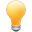Follow us on our fanpages to receive notifications every time there are new articles.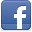Facebook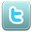Twitter

1- Introduction

This is guide of C# for beginners. In order to program C# you must install Visual Studio programming tools. You can see the instructions to download and install at:
If you are new to C#, you should read this document from beginning to end, which will give you an overview before learning other detailed documents.

2- Create your first C# project

This is the first image when you open Visual Studio.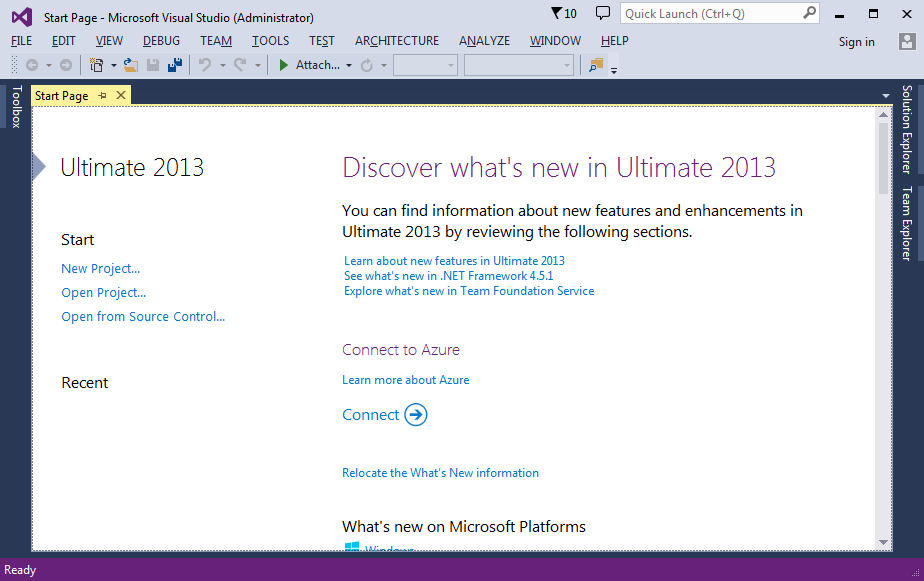Create new Project: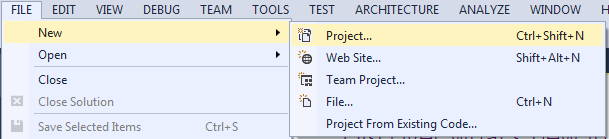We created a simple Project (Console application, an application has no interface). Enter:
• Name: HelloCSharp
• Solution: Create new solution
• Solution Name: MySolution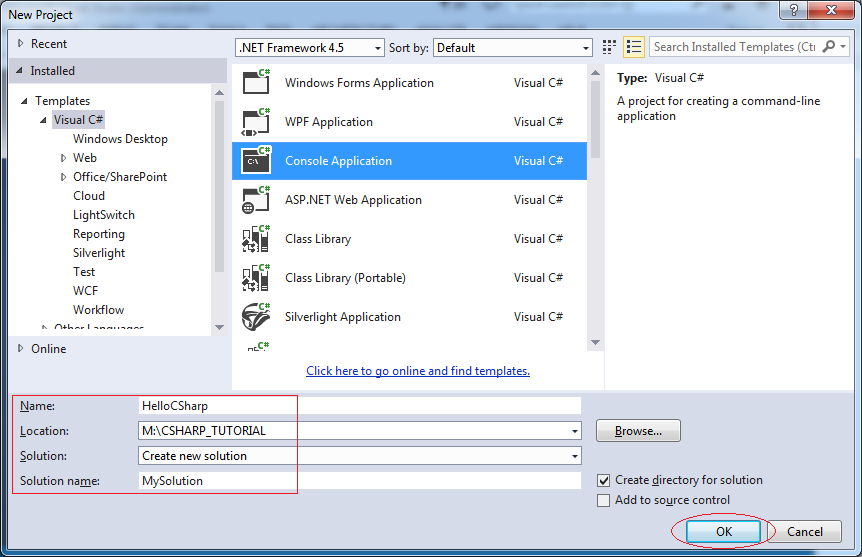This is an image of your Project that was created. You need to click on the Solution Explorer to view the structure of this created Project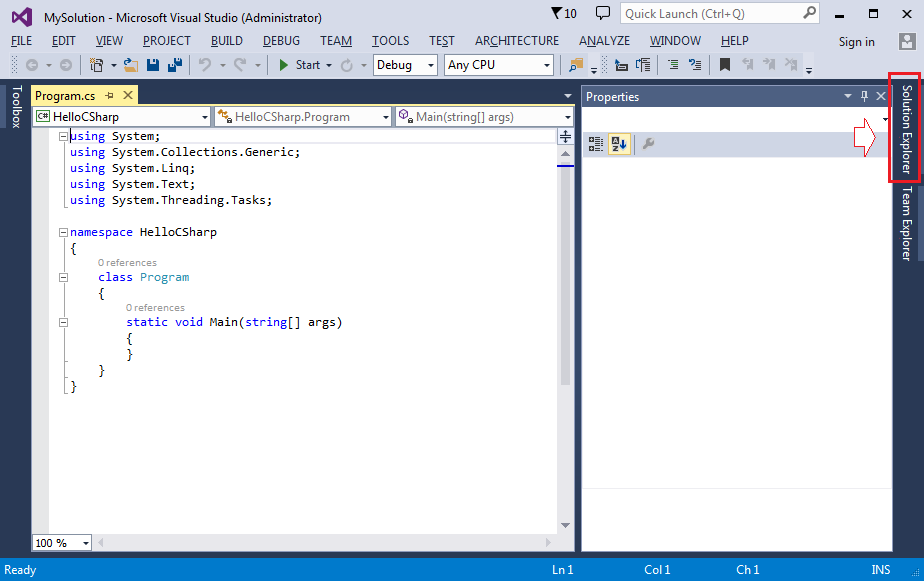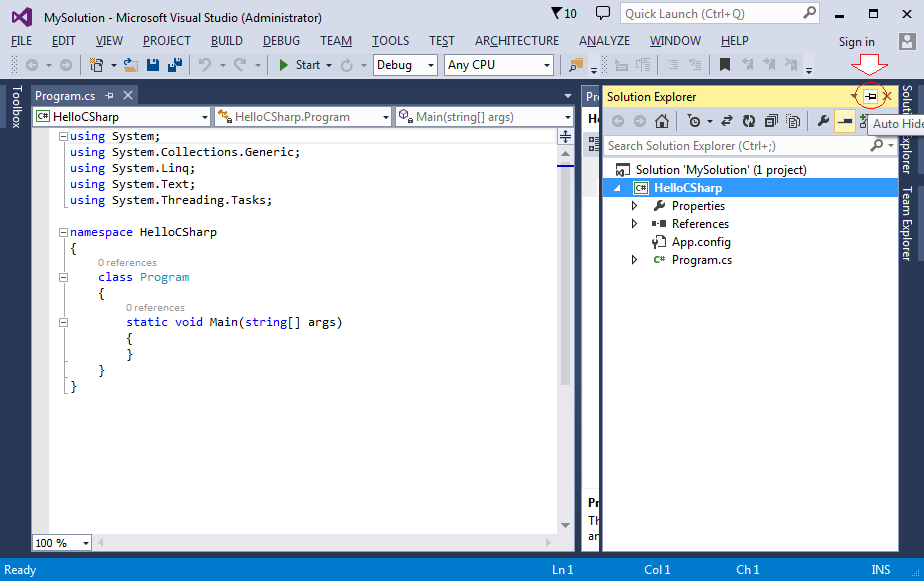Visual Studio creates a Solution, which is named MySolution and contained  a Project named HelloCSharp. And create by default a class named Program (corresponding to Program.cs file).
Note: A Solution can have one or several  Projects.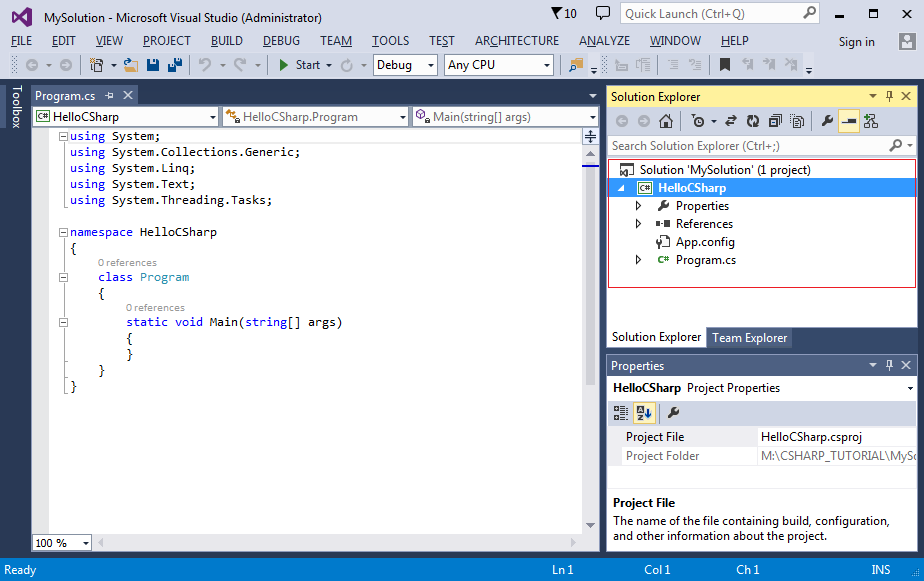Edit code of Program class, so when running, it will display on the Console screen "Hello CSharp", and waiting the user enters any text before finishing.
Click Start to run Program class.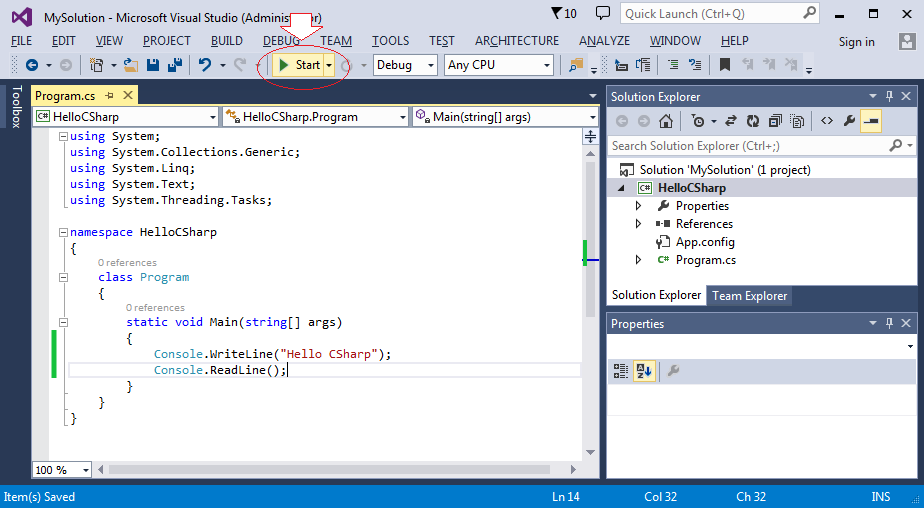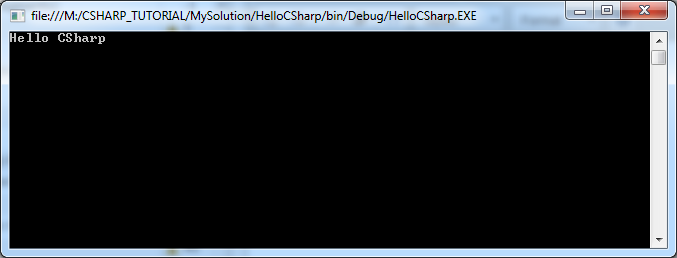3- Explain the structure of a class

The illustration below is the structure of the Program class , which is located in the HelloCSharp namespace . A namespace can contain one or several classes.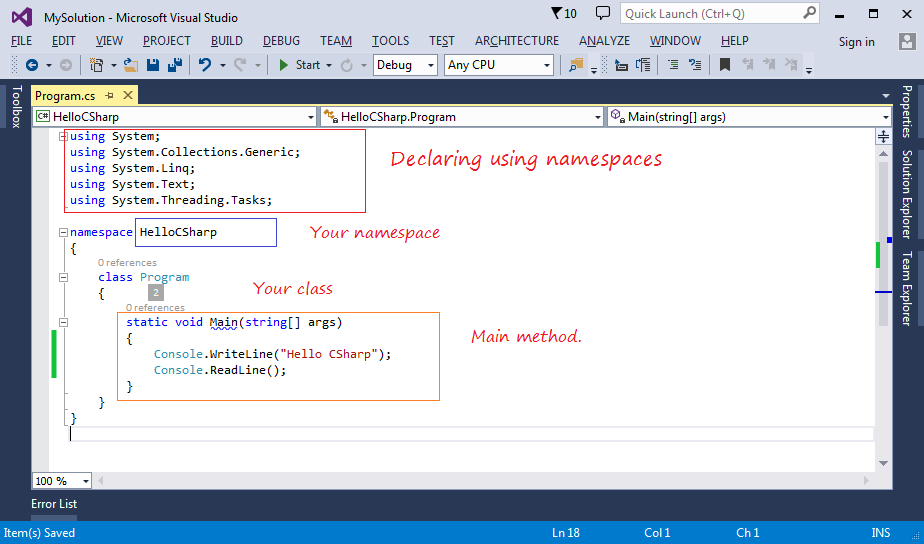If you want to use a certain class, you must declare to use this class, or declare to use namespace that contains this class.

// Declaring to use System namespace.
// (That means you can use all the classes,.. in this namespace).
using System;
When the program runs, the Main(string[]) method will be called to execute.
1. static is the keyword informed that it is a static method.
2. void  is the key word informed that the method does not return anything.
3. args is parameter of the method, an array of strings - string[].

static void Main(string[] args)
{
// Print text to the console window.
Console.WriteLine("Hello CSharp");

// Wait for the user to enter something and press Enter before ending the program.
}
After declaring the use of the System namespace, you can use the Console class in this namespace. WriteLine(string) is a static method of Console  class, it print to screen a string.

// Declare to use System namespace
// (It contains the Console class).
using System;

// And can use the Console class:
Console.WriteLine("Hello CSharp");

// If you do not want to declare System namespace.
// But want to use Console class, you must write the full:
System.Console.WriteLine("Hello CSharp");

4- Explaining structure of Project

A solution may contain within it many Projects. A Project contains the classes.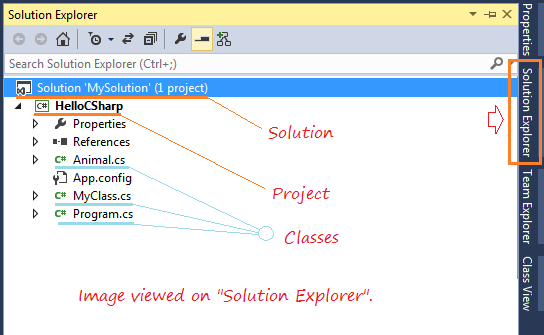When viewing in "Class view" you can see your classes belong to which namespace.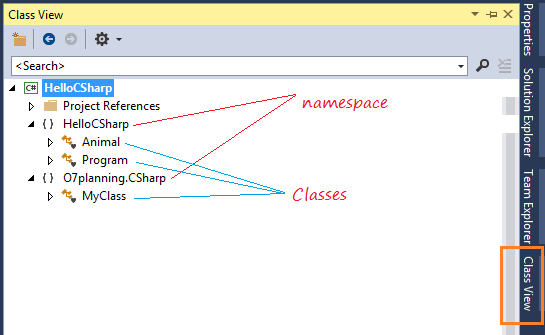In CSharp, you create an Animal class with namespace named HelloCSharp, this class will be located in the  root directory of the project by default. You create another class is MyClass with namespace named  O7planning.CSharp class also located in the root directory of the project. With a large project with many classes, organize the files as such make it difficult for you. You can create different folders to store class files, the rule is your decision, but it's best to create a folder named as the name of the namespace. Let's learn how to organize of Java.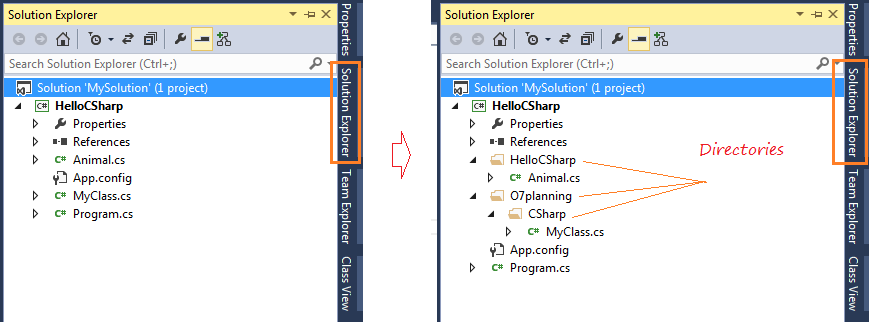5- Important note with C# program

In C# application, you need to explicitly declare a class with the  Main(string[]) method as a starting point to run your application, this is not compulsory if your entire application have only one class with the Main(string[]) method, however, in case you have 2 classes with the Main method, if you do not specify, an error message will appear in compilation process.

Therefore,  you should preferably  declare clearly the class with the  Main(string[]) method, you can declare again for another class if desired.
Right-click the HelloCSharp project, select Properties: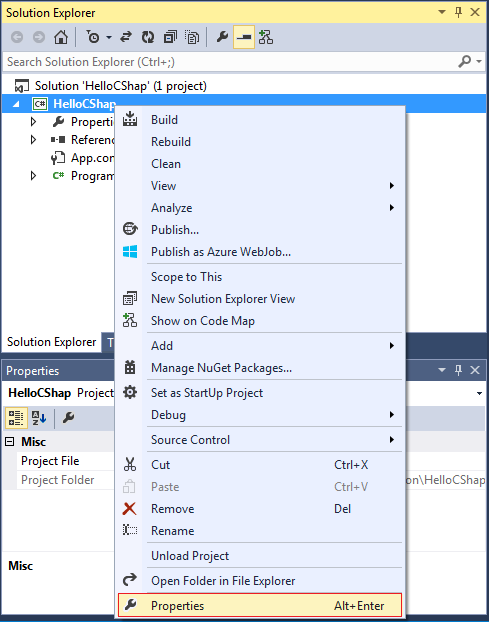Select the "Startup object" is a class have Main(string[]) method, and Save.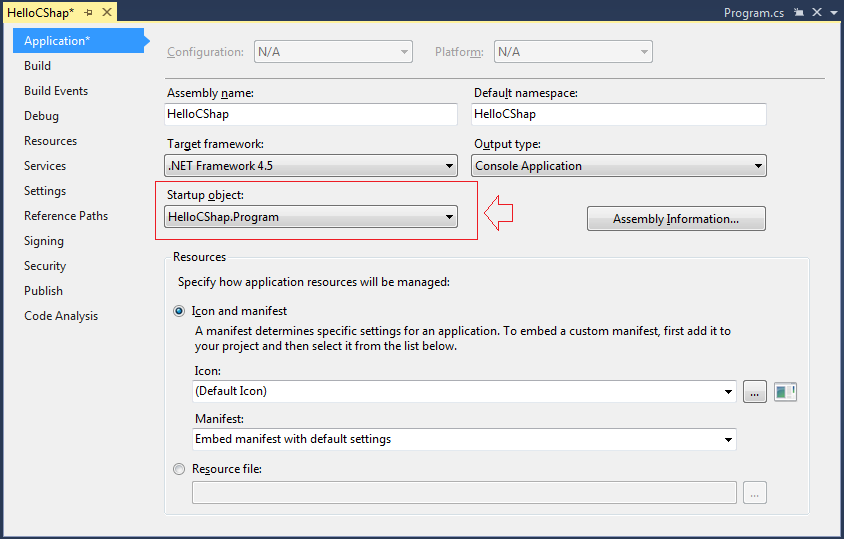Now I add a MyClass class with namespace named MyNamespace.Abc.
In "Solution Explorer" right-click the project, select: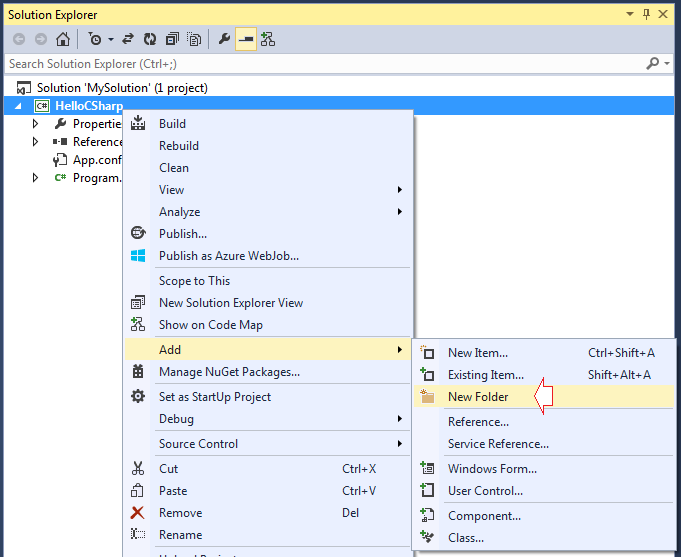Name the folder is O7planning.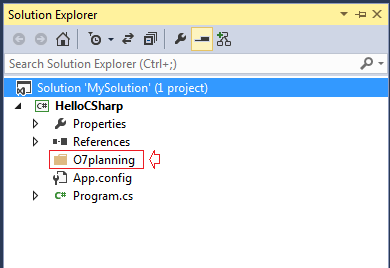Next, create a folder "CSharp" is a subfolder of "O7planning".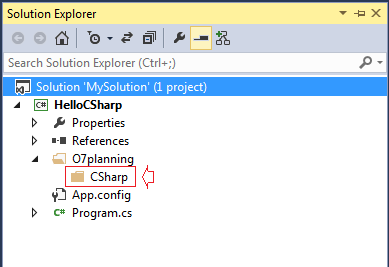Right-click on the "CSharp" folder, select: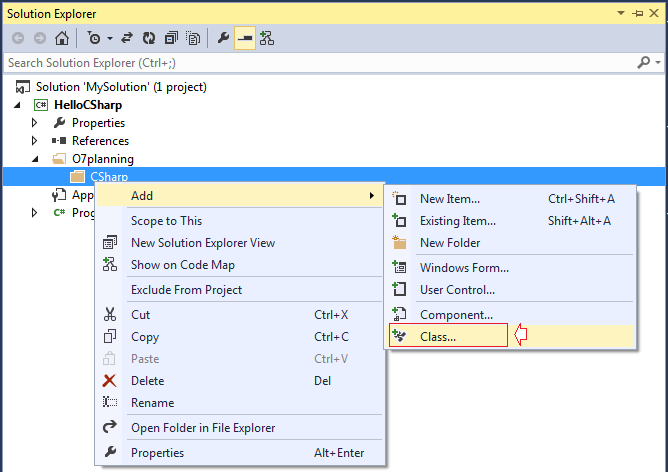Select the type of item is Class, and enter the class name.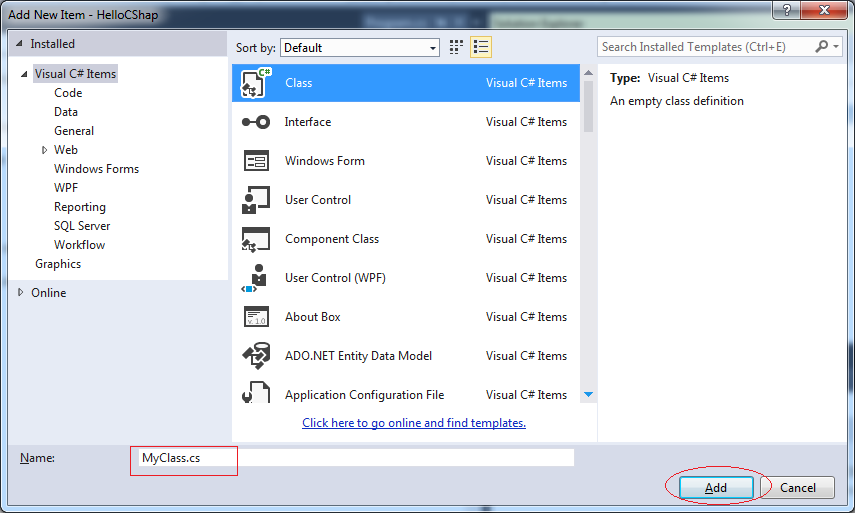Class has been created, it is located in "Hello CSharp.O7planning.CSharp" namespace. You can rename to "O7planning.CSharp".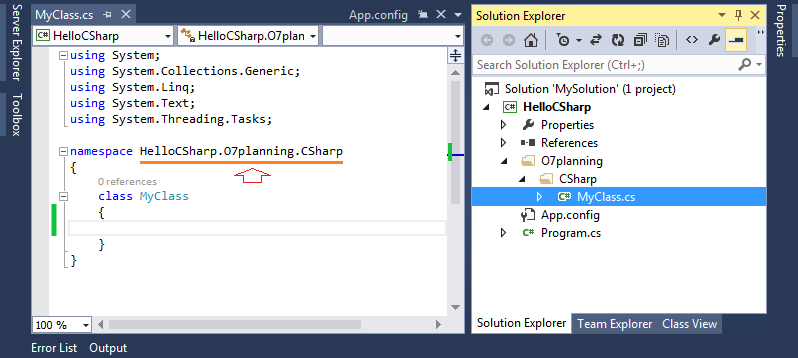Rename namespace to "O7planning.CSharp".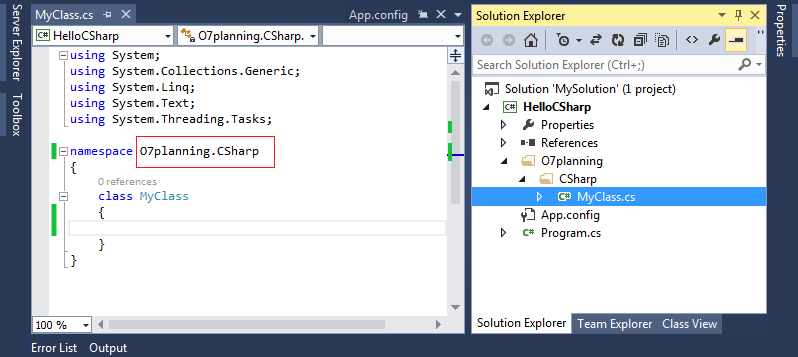You can change content of the class:
MyClass.cs

using System;
using System.Collections.Generic;
using System.Linq;
using System.Text;

namespace O7planning.CSharp
{
class MyClass
{
static void Main(string[] args)
{
Console.WriteLine("Hello from MyClass");
}
}
}
Declaring class MyClass is the starting point to run. Right click Project and Select Properties.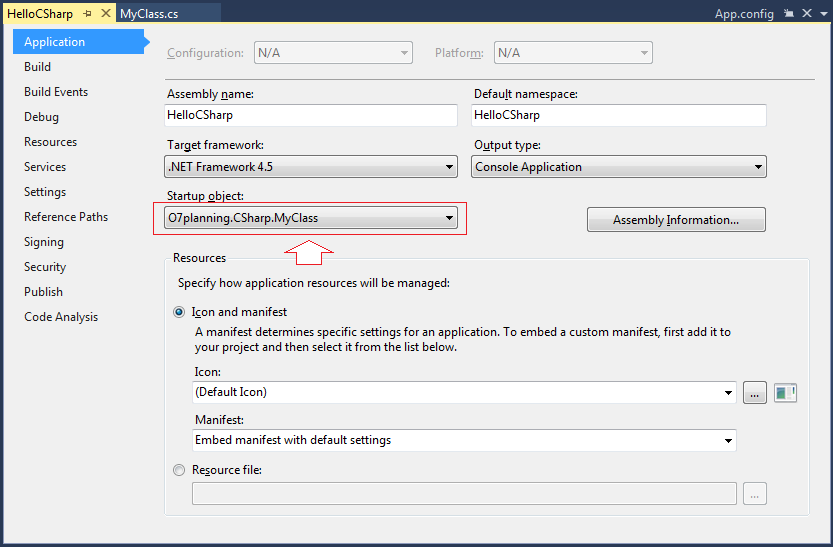Running the example: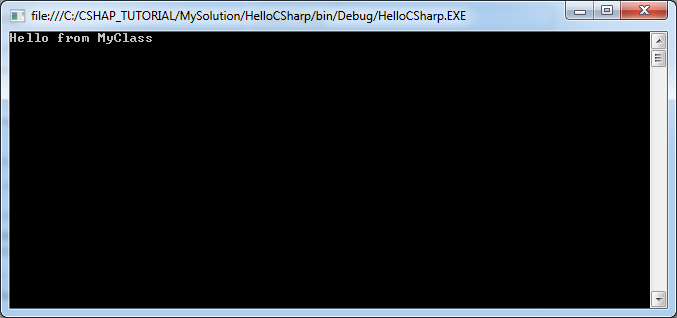7- Data types in C #

Type Represents Range Default Value
bool Boolean value True or False False
byte 8-bit unsigned integer 0 to 255 0
char 16-bit Unicode character U +0000 to U +ffff '\0'
decimal 128-bit precise decimal values with 28-29 significant digits (-7.9 x 1028 to 7.9 x 1028) / 100 to 28 0.0M
double 64-bit double-precision floating point type (+/-)5.0 x 10-324 to (+/-)1.7 x 10308 0.0D
float 32-bit single-precision floating point type -3.4 x 1038 to + 3.4 x 1038 0.0F
int 32-bit signed integer type -2,147,483,648 to 2,147,483,647 0
long 64-bit signed integer type -923,372,036,854,775,808 to 9,223,372,036,854,775,807 0L
sbyte 8-bit signed integer type -128 to 127 0
short 16-bit signed integer type -32,768 to 32,767 0
uint 32-bit unsigned integer type 0 to 4,294,967,295 0
ulong 64-bit unsigned integer type 0 to 18,446,744,073,709,551,615 0
ushort 16-bit unsigned integer type 0 to 65,535 0

8- Variables and variable declaration

A variable is identified by a name, for a data storage area that your program can be manipulated. Each variable in C# has a specific type, which determines the size and layout of the variable's memory the range of values that can be stored within that memory and the set of operations that can be applied to the variable.
Variable can change value in the course of its existence in the program. The variables have a fixed value called constants. Using the const keyword to declare a  constant variable.
Declare a variable:

// Declare a variable.
<Data Type> <Variable name>;

// Declares a variable, and assign a value to it.
<Data Type> <Variable name> = <value>;

// Declare a constant:
const <Data Type> <Variable name> = <value>;
VariableExample.cs

using System;
using System.Collections.Generic;
using System.Linq;
using System.Text;

namespace HelloCSharp
{
class VariableExample
{
static void Main(string[] args)
{
// Declare a constant integer.
// You can not assign new values for the constants.
const int MAX_SCORE = 100;

// Declare an integer variable
int score = 0;

// Assign a new value for the variable score.
score = 90;

// Declare a string
string studentName = "Tom";

// Print value of variable to the Console.
Console.WriteLine("Hi {0}", studentName);

// Wait for the user to enter something and press Enter before ending the program.
}

}
}
Running the example: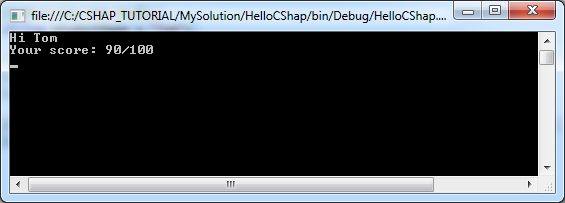9- Branch Statements

9.1- If-else Statement

if is a statement which checks a certain condition in C#. For example: If a> b, then do something ....
The common comparison operators:
 Operator Meaning Example > greater than 5 > 4 is true < less than 4 < 5 is true >= greater than or equal 4 >= 4 is true <= less than or equal 3 <= 4 is true == equal to 1 == 1 is true != not equal to 1 != 2 is true

// Syntax:
//
if ( condition )
{
// Do somthing here.
}
Example:

// Example 1:
if ( 5 < 10 )
{
Console.WriteLine( "Five is now less than ten");
}

// Example 2:
if ( true )
{
Console.WriteLine( "Do something here");
}
Full structure of the if - else if - else:

// Note that at most one block of code will be executed.
// The program checks the conditions from top to bottom.
// When a condition is true, this block will be executed.
// The remaining conditions will be ignored.
...

// If condition1 is true then ..
if (condition1 )
{
// Do something if condition1 is true.
}
// Else if condition2 is true then ....
else if(condition2 )
{
// Do something here if condition2 is true (condition1 is false).
}
// Else if conditionN is true then ...
else if(conditionN )
{
// Do something if conditionN is true
// (All the above conditions are false)
}
// Else (All the above conditions are false).
else  {
//  Do something.
}
IfElseExample.cs

using System;
using System.Collections.Generic;
using System.Linq;
using System.Text;

namespace HelloCSharp
{
class IfElseExample
{
static void Main(string[] args)
{
// Declare an integer describes your age.
int age;

// Declare a variable to store the user input from the keyboard.

// Int32 is a class in the System namespace.
// Using static method Int32.Parse to convert a string to number
// and assign to 'age'.
// (Note: If 'inputStr' is not a number, error will be thrown).
age = Int32.Parse(inputStr);

// Check if age less than 80 then ...
if (age < 80)
{
Console.WriteLine("You are pretty young");
}

// Else if age between 80 and 100 then
else if (age >= 80 && age <= 100)
{
Console.WriteLine("You are old");
}
// Else (The remaining cases)
else
{
Console.WriteLine("You are verry old");
}

}
}
}
Running example, and entering 81, and see the results: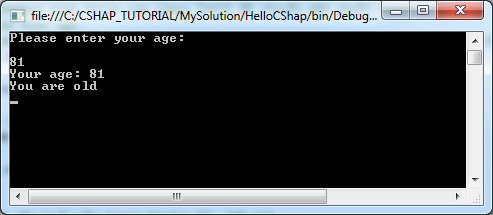9.2- Switch-Case Statement

Syntax of switch:

// Use the 'switch' to check the value of a variable.
switch ( <variable> )
{
case value1:
// Do something if the value of the variable equal to value1.
break;
case value2:
// Do something if the value of the variable equal to value2.
break;

...

default:
// The remaining cases.
break;
}
BreakExample.cs

using System;
using System.Collections.Generic;
using System.Linq;
using System.Text;

namespace HelloCSharp
{
class BreakExample
{
static void Main(string[] args)
{
// Require users to select an option.

Console.WriteLine("1 - Play a game \n");
Console.WriteLine("2 - Play music \n");
Console.WriteLine("3 - Shutdown computer \n");

// Declare a variable 'option'.
int option;

// The string that the user entered from the keyboard

// Convert to integer.
option = Int32.Parse(inputStr);

// Check the value of 'option'.
switch (option)
{

case 1:
Console.WriteLine("You choose to play the game");
break;
case 2:
Console.WriteLine("You choose to play the music");
break;
case 3:
Console.WriteLine("You choose to shutdown the computer");
break;
default:
Console.WriteLine("Nothing to do...");
break;
}

}
}
}
Running the example, and entering 2: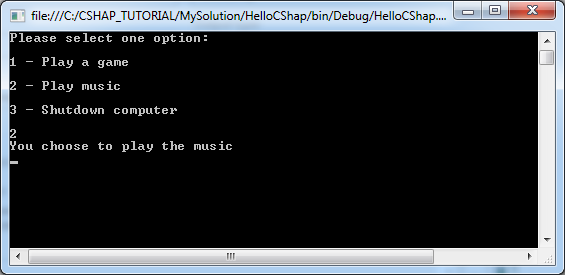In this case, break told the program to exit switch.

You can include many "case" together to execute a same block.
BreakExample2.cs

using System;
using System.Collections.Generic;
using System.Linq;
using System.Text;

namespace HelloCSharp
{
class BreakExample2
{
static void Main(string[] args)
{
// Declare a varable and assign value of 3.
int option = 3;

Console.WriteLine("Option = {0}", option);

// Check value of 'option'.
switch (option)
{

case 1:
Console.WriteLine("Case 1");
break;

// If value in (2,3,4,5)
case 2:
case 3:
case 4:
case 5:
Console.WriteLine("Case 2,3,4,5!!!");
break;
default:
Console.WriteLine("Nothing to do...");
break;
}

}
}
}
Running the example: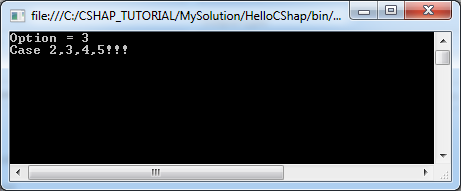10- The loop in C #

The loop is used to run repetitively a statement block . It makes your program execute repeatedly a statement block several times, this is one of the basic tasks of programming.
C# supports three different kinds of loops:
• FOR
• WHILE
• DO WHILE

10.1- for loop

The syntax of the for loop:

for (initialize variable ; condition ; updates new value for variable )
{
// Execute the block when the condition is true
}
Example:

// Example 1:
// Declare a variable x and assign value 0.
// The condition is x < 5
// If x < 5 is true then execute the block.
// After each iteration, the value of x is increased by 1.
for (int  x = 0;  x < 5 ; x = x + 1)
{
// Do something here when x < 5 is true.
}

// Example 2:
// Declare variable x and assgin value of 2
// The condition is x < 15
// If x < 15 is true then execute the block.
// After each iteration, the value of x is increased by 3.
for (int  x = 2;  x < 15 ; x = x + 3)
{
// Do something here when x < 15 is true.
}
ForLoopExample.cs

using System;
using System.Collections.Generic;
using System.Linq;
using System.Text;

namespace HelloCSharp
{
class ForLoopExample
{
static void Main(string[] args)
{
Console.WriteLine("For loop example");

// Declare variable x and assign value of 2.
// The condition is x < 15
// If x < 15 is true then execute the block.
// After each iteration, the value of x is increased by 3
for (int x = 2; x < 15; x = x + 3)
{
Console.WriteLine( );
Console.WriteLine("Value of x = {0}", x);
}

}
}
}
Running the example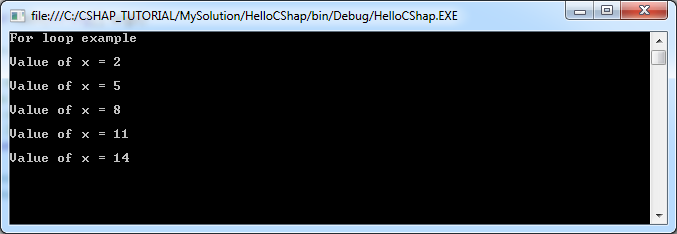10.2- while loop

Syntax of while loop:

while (condition)
{
// While the 'condition' is true, then execute the block.
}
Example:

// Declare a variable x.
int x = 2;

while ( x < 10)
{
// Do something here when x < 10 is true.
...

// Update value to x.
x = x + 3;
}
WhileLoopExample.cs

using System;
using System.Collections.Generic;
using System.Linq;
using System.Text;

namespace HelloCSharp
{
class WhileLoopExample
{
static void Main(string[] args)
{

Console.WriteLine("While loop example");

// Declare a variable x and assign value of 2.
int x = 2;

// The condition is x < 10.
// If x < 10 is true then execute the block.
while (x < 10)
{
Console.WriteLine("Value of x = {0}", x);

x = x + 3;
}

}
}
}
Running the example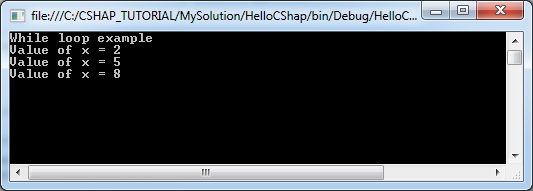10.3- do-while loop

Syntax of do-while loop

// Characteristics of the 'do-while' loop is that it will execute the block at least 1 time.
// After each iteration, it will check condition,
// If condition is true, the block will be executed.
do {
// Do something here.
} while (condition);
// Note: it should have a semicolon here.
DoWhileLoopExample.cs

using System;
using System.Collections.Generic;
using System.Linq;
using System.Text;

namespace HelloCSharp
{
class DoWhileLoopExample
{
static void Main(string[] args)
{

Console.WriteLine("Do-While loop example");

// Declare a variale x and assign value of 2
int x = 2;

// Execute the block.
// After each iteration, it will check condition,
// If condition is true, the block will be executed.
do
{
Console.WriteLine("Value of x = {0}", x);

x = x + 3;

} while (x < 10);
// Note: it should have a semicolon here.
}
}
}
Running the example: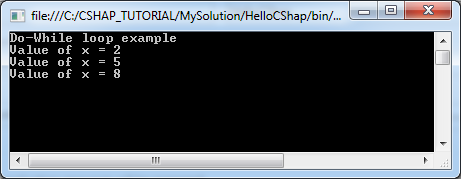10.4- The break in the loop

break is a command that may be located in a block of a loop. This command ends the loop unconditionally.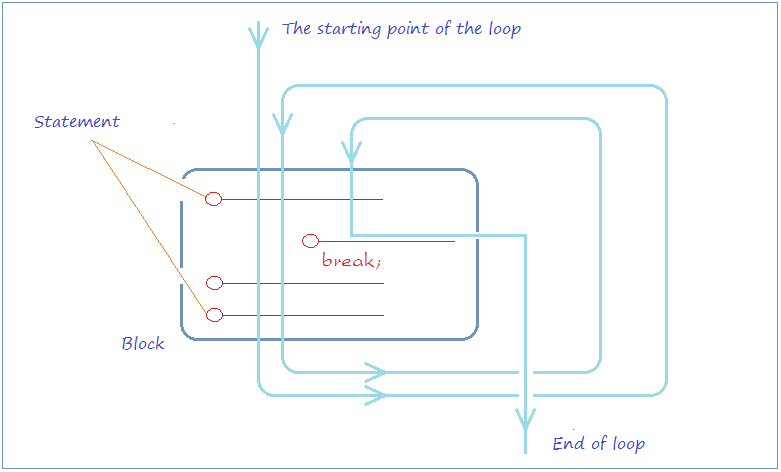LoopBreakExample.cs

using System;
using System.Collections.Generic;
using System.Linq;
using System.Text;

namespace HelloCSharp
{
class LoopBreakExample
{
static void Main(string[] args)
{
Console.WriteLine("Break example");

// Declare a variable and assign value of 2
int x = 2;

while (x < 15)
{

Console.WriteLine("----------------------\n");
Console.WriteLine("x = {0}", x);

// If x = 5 then exit the loop.
if (x == 5)
{
break;
}
// Increase value of x by 1 (Write brief x = x + 1;).
x++;
Console.WriteLine("x after ++ = {0}", x);

}

}
}
}
Running the example: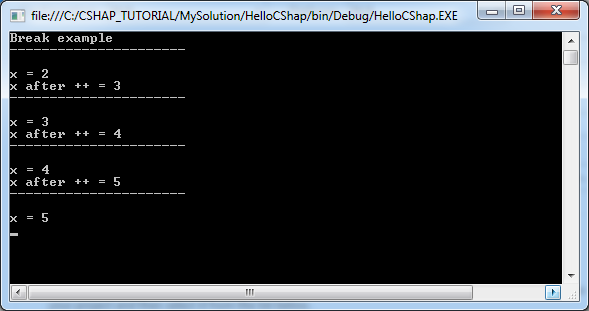10.5- Continue statement within the loop

continue is a statement which may be located in a loop. When caught the continue statement, the program will ignore the command lines in block, below of continue and start of a new loop.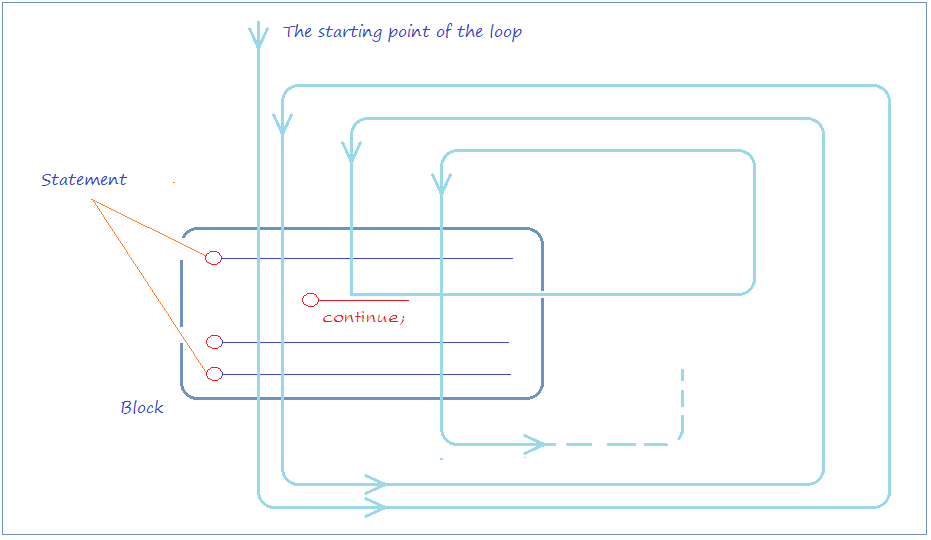LoopContinueExample.cs

using System;
using System.Collections.Generic;
using System.Linq;
using System.Text;

namespace HelloCSharp
{
class LoopContinueExample
{
static void Main(string[] args)
{

Console.WriteLine("Continue example");

// Declare a variable and assign value of 2.
int x = 2;

while (x < 7)
{

Console.WriteLine("----------------------\n");
Console.WriteLine("x = {0}", x);

// % operator is used for calculating remainder.
// If x is even, then ignore the command line below of 'continue',
// and start new iterator (if condition still true).
if (x % 2 == 0)
{
// Increase x by 1. (write brief for x = x + 1;).
x++;
continue;
}
else
{
// Increase x by 1. (write brief for x = x + 1;).
x++;
}
Console.WriteLine("x after ++ = {0}", x);

}

}
}
}
Running the example: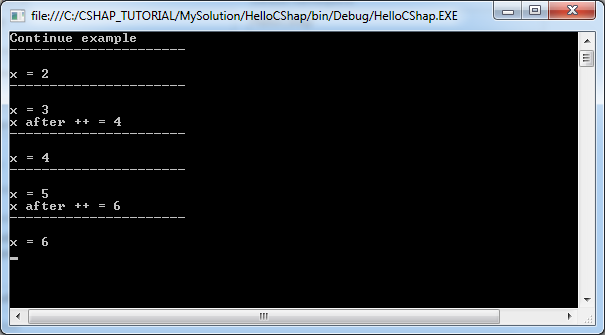11- Arrays in C #

11.1- One-dimensional array

These are illustrations of one dimension array with 5 elements which are indexed from 0 to 4.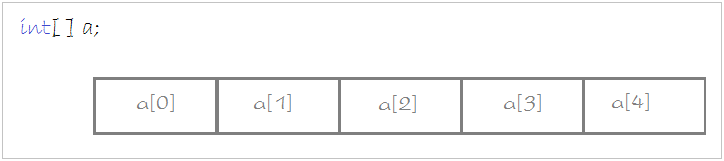The syntax to declare a one-dimensional array:

// Way 1:
// Declare an array of int, specifies the elements.
int[] years = { 2001, 2003, 2005, 1980, 2003 };

// Way 2:
// Declare an array of floats, specifying the number of elements.
// (3 elements).
float[] salaries = new float;
ArrayExample1.cs

using System;
using System.Collections.Generic;
using System.Linq;
using System.Text;

namespace HelloCSharp
{
class ArrayExample1
{
static void Main(string[] args)
{
// Way 1:
// Declare an array of int, specifies the elements.
int[] years = { 2001, 2003, 2005, 1980, 2003 };

// Length is a property of the array, it returns the number of elements.
Console.WriteLine("Element count of array years = {0} \n", years.Length);

// Use a for loop to print out the elements of the array.
for (int i = 0; i < years.Length; i++) {
Console.WriteLine("Element at {0} = {1}", i, years[i]);
}

// Way 2:
// Declare an array of floats, specifying the number of elements.
// (3 elements).
float[] salaries = new float;

// Assign values to the elements.
salaries = 1000;
salaries = 1200;
salaries = 1100;

}
}
}
Running the example: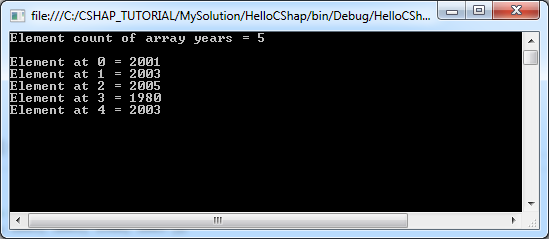11.2- Two-dimensional array

This is the illustration of a 2-dimensional array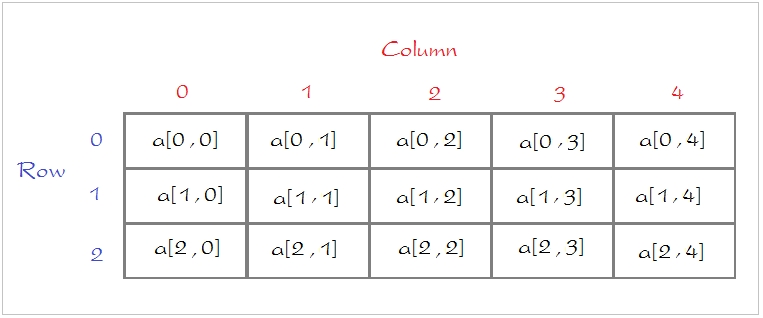The syntax to declare a two-dimensional array:

// Declare 2-dimensional array, 3 rows & 5 columns.
// specify the elements.
int[,] a =   new int[,]  {
{1 , 2 , 3,  4,   5} ,
{0,  3,  4,  5,   7},
{0,  3,  4,  0,   0}
};

// Declare a 2-dimensional array, 3 rows & 5 columns
// The element has not been assigned a value.
int[,] a = new int[3,5];
ArrayExample2.cs

using System;
using System.Collections.Generic;
using System.Linq;
using System.Text;

namespace HelloCSharp
{
class ArrayExample2
{
static void Main(string[] args)
{
// Declare 2-dimensional array, 3 rows & 5 columns.
// Specify the elements.
int[,] a =   {
{ 1, 2, 3, 4, 5 },
{ 0, 3, 4, 5, 7 },
{ 0, 3, 4, 0, 0 }
};

// Use a for loop to print out the elements of the array.
for (int row = 0; row < 3; row++) {
for (int col = 0; col < 5; col++) {
Console.WriteLine("Element at [{0},{1}] = {2}", row, col, a[row,col]);
}
Console.WriteLine("-------------");
}

// Declare a 2-dimensional array, 3 rows, 5 columns
// The element has not been assigned a value.
int[,] b = new int[3, 5];

}
}
}
Running the example: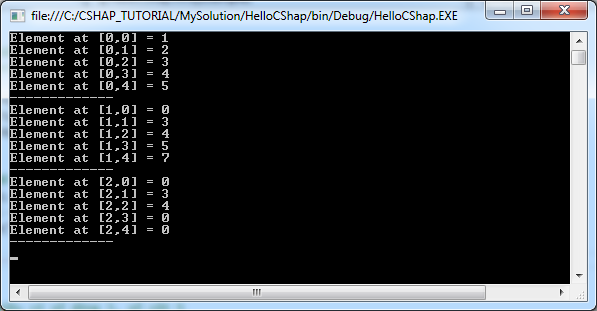11.3- Array of an array

ArrayOfArrayExample.cs

using System;
using System.Collections.Generic;
using System.Linq;
using System.Text;

namespace HelloCSharp
{
class ArrayOfArrayExample
{
static void Main(string[] args)
{
// Declare an array of 3 elements.
// Each element is another array.
string[][] teams = new string[];

string[] mu = { "Beckham","Giggs"};
string[] asenal = { "Oezil", "Szczęsny", "Walcott" };
string[] chelsea = {"Oscar","Hazard","Drogba" };

teams = mu;
teams = asenal;
teams = chelsea;

// Use a for loop to print out the elements of the array.
for (int row = 0; row < teams.Length; row++)
{
for (int col = 0; col < teams[row].Length ; col++)
{
Console.WriteLine("Element at [{0}],[{1}] = {2}", row, col, teams[row][col]);
}
Console.WriteLine("-------------");
}

}
}
}
Running the example: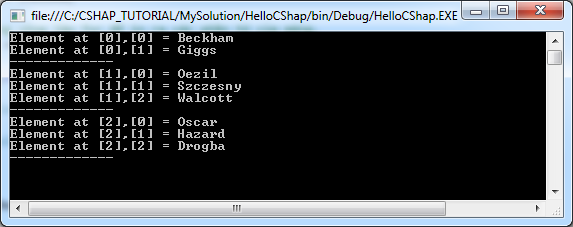12- Class, constructor and instance

You need to have a distinction between three concepts:
• Class
• Constructor
• Instance
When we talk about the tree, it is something abstract, it is a class. But when we pointed to a specific tree, it was clear, and that is the instance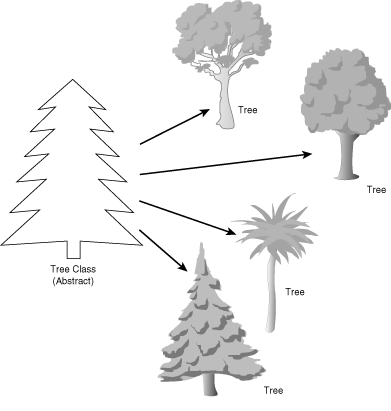Or when we talk about the Person, that's abstract, it is a class. But when pointed at you or me, it is two different instances, the same class of People.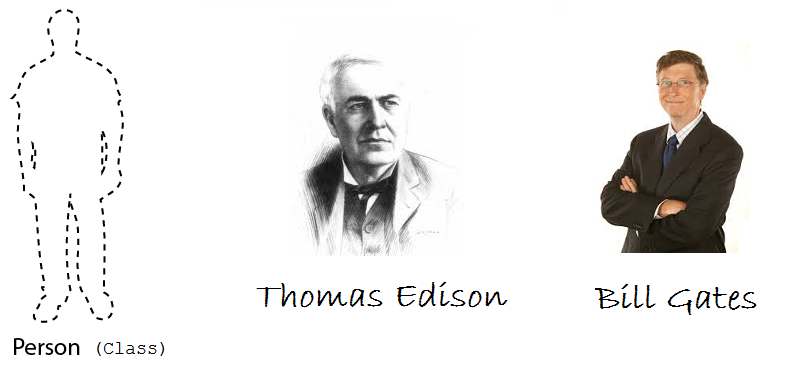Person.cs

using System;
using System.Collections.Generic;
using System.Linq;
using System.Text;

namespace HelloCSharp
{
class Person
{
// This is a Field.
// To store name of Person.
public string Name;

// This is a Constructor,
// Used to initialize the object.
// This constituent has one parameter.
// Constructor always have the same name as the class
public Person(string persionName)
{
// Assign value of parameter to field 'Name'.
this.Name = persionName;
}

// This is a method return type of string.
public string GetName()
{
return this.Name;
}
}
}
Person class without Main method. Next in PersonTest, we create Person instance from its Constructor
PersonTest.cs

using System;
using System.Collections.Generic;
using System.Linq;
using System.Text;

namespace HelloCSharp
{
class PersonTest
{
static void Main(string[] args)
{
// Create an object of Person class
// Initialize this object from constructor of Person class.
Person edison = new Person("Edison");

// Person class has GetName() method.
// Using object to call GetName() method.
String name = edison.GetName();
Console.WriteLine("Person 1: " + name);

// Create object of Person class.
// Initialize this object from Constructor of Person class.
Person billGate = new Person("Bill Gates");

// Access the public field of Person.
String name2 = billGate.Name;
Console.WriteLine("Person 2: " + name2);

}
}
}
Running the example: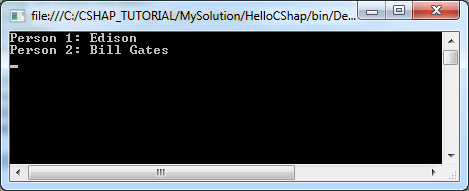13- Field

In this section we will discuss some of the concepts:
• Field
• Normal field
• static Field
• const Field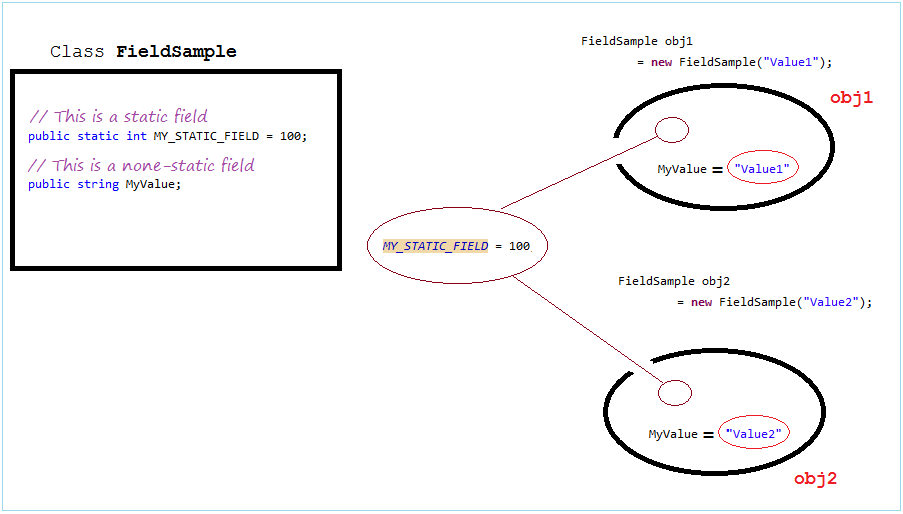FieldSample.cs

using System;
using System.Collections.Generic;
using System.Linq;
using System.Text;

namespace HelloCSharp
{
class FieldSample
{
// This is static field.
public static int MY_STATIC_FIELD = 100;

// This is a field.
public string MyValue;

// Constructor of FieldSample class.
public FieldSample(string value)
{
this.MyValue = value;
}
}
}
FieldSampleTest.cs

using System;
using System.Collections.Generic;
using System.Linq;
using System.Text;

namespace HelloCSharp
{
class FieldSampleTest
{
static void Main(string[] args)
{
// Print out value of static field.
// With the static field, you must access it through the class.
Console.WriteLine("FieldSample.MY_STATIC_FIELD= {0}", FieldSample.MY_STATIC_FIELD);

// Change value of static field
FieldSample.MY_STATIC_FIELD = 200;

Console.WriteLine(" ------------- ");

// Create first object.
FieldSample obj1 = new FieldSample("Value1");

// With the none-static field, you must access it through object.
Console.WriteLine("obj1.MyValue= {0}",  obj1.MyValue);

// Create second object.
FieldSample obj2 = new FieldSample("Value2");

Console.WriteLine("obj2.MyValue= {0}" , obj2.MyValue);

// You can change value of field.
obj2.MyValue = "Value2-2";

}

}
}
Running the example: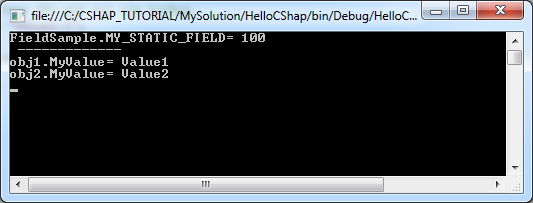ConstFieldExample.cs

using System;
using System.Collections.Generic;
using System.Linq;
using System.Text;

namespace HelloCSharp
{
class ConstFieldExample
{
// A constant field, its value is determined at compile time.
// You can not assign new values for the constants
// NOTE: constant is always static.
public const int MY_VALUE = 100;

// A static field and readonly.
// Its value is assigned once at the time of the first run.
public static readonly DateTime INIT_DATE_TIME1 = DateTime.Now;

// A readonly and none-static field.

public ConstFieldExample()
{
// Its value is assigned once at the time of the first run.
INIT_DATE_TIME2 = DateTime.Now;
}
}
}

14- Method

Method
• Method.
• static Method
• sealed Method. (Will be mentioned in the inheritance of the class).
MethodSample.cs

using System;
using System.Collections.Generic;
using System.Linq;
using System.Text;

namespace HelloCSharp
{
class MethodSample
{
public string text = "Some text";

// Default constructor.
// (Constructor with no parameters).
public MethodSample()
{

}

// This is a method return string.
// This method with no parameter.
public string GetText()
{
return this.text;
}

// This is a method with one String parameter.
// Return void (return nothing).
public void SetText(string text)
{
// this.text: point to 'text' field.
this.text = text;
}

// This is static method
// return int and has 3 parameters.
public static int Sum(int a, int b, int c)
{
int d = a + b + c;
return d;
}
}
}
MethodSampleTest.cs

using System;
using System.Collections.Generic;
using System.Linq;
using System.Text;

namespace HelloCSharp
{
class MethodSampleTest
{
static void Main(string[] args)
{
// Create MethodSample object.
MethodSample obj = new MethodSample();

// Call GetText() method.
// The non-static method must be called through the object.
String text = obj.GetText();

Console.WriteLine("Text = " + text);

// Call SetText(string) method.
// The non-static method must be called through the object.
obj.SetText("New Text");

Console.WriteLine("Text = " + obj.GetText());

// The static method must be called through the class.
int sum = MethodSample.Sum(10, 20, 30);

Console.WriteLine("Sum  10,20,30= " + sum);

}
}
}
Running the example: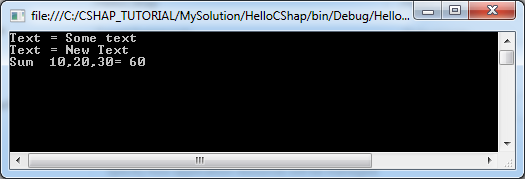15- Inheritance in C#

Java allows classes which extend from other class. Class extends another class called subclasses. Subclasses have the ability to inherit the fields attributes, methods from the parent class.
See an example of inheritance in CSharp:
Animal.cs

using System;
using System.Collections.Generic;
using System.Linq;
using System.Text;

namespace HelloCSharp
{
// Animal
class Animal
{
public Animal()
{

}

public void Move()
{
Console.WriteLine("Move ...!");
}

}
}
Cat.cs

using System;
using System.Collections.Generic;
using System.Linq;
using System.Text;

namespace HelloCSharp
{
class Cat : Animal
{

public   void Say()
{
Console.WriteLine("Meo");
}

// A method of Cat.
public void Catch()
{
Console.WriteLine("Catch Mouse");
}
}
}
Ant.cs

using System;
using System.Collections.Generic;
using System.Linq;
using System.Text;

namespace HelloCSharp
{
// Ant
class Ant : Animal
{
}
}
AnimalTest.cs

using System;
using System.Collections.Generic;
using System.Linq;
using System.Text;

namespace HelloCSharp
{
class AnimalTest
{

static void Main(string[] args)
{
// Create Cat object.
Cat tom = new Cat();

// Check if 'tom' is Animal
// The result is true
bool isAnimal = tom is Animal;

// ==> true
Console.WriteLine("tom is Animal? " + isAnimal);

// Call Catch method.
tom.Catch();

// Call Say() method
// ==> Meo
tom.Say();

Console.WriteLine("--------------------");

// Declare an Animal object.
// Create it from Constructor of Cat.
Animal tom2 = new Cat();

// Call Move() method.
tom2.Move();

Console.WriteLine("--------------------");

// Create an Ant object.
Ant ant = new Ant();

// Call Move() method, inherited from Animal.
ant.Move();

}
}
}
Running the example: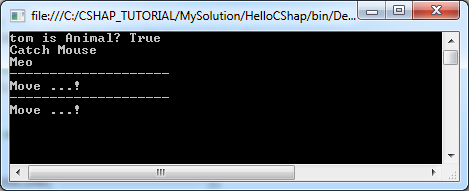16- Inheritance and polymorphism in C#

You can read more "Inheritance and polymorphism in CSharp" at:

View more Tutorials:

Maybe you are interested

These are online courses outside the o7planning website that we introduced, which may include free or discounted courses.

•Learn and Understand Interfaces in C#
•Design Patterns in C# and .NET
•C# Basics - Learn to Code the Right Way
•Complete C++, C# and C programming tools for complete NOVICE
•A beginners guide to C# Scripting for Unity Game Development
•Learn to code by creating 14 projects with JavaScript and C#
•Intro to C# Programming and Scripting for Games in Unity
•Xamarin Android - A Master Guide to App Development in C#
•Learn C# From Scratch with Real Applications
•C# Design patterns
•Starting 2D Game Development in Unity with C#
•The complete algorithms course in C# and .Net
•Complete C# Unity Developer 2D: Learn to Code Making Games
•Naked C#: A Beginner's Guide to Coding
•Intro to C# Programming and Scripting for Games in Unity
•Learn ASP NET with Bootstrap,Entity Framework,JavaScript,C#
•Internet of things development for C# programmers
•C# Basics - Learn Coding & Programming for Beginners
•An Introduction to C# for the Rest of Us
•C# Beginner To Professional Software Developer
•C# Loops for Beginners
•What's New in C#7 and C# 8
•ASP NET Core 3 (ASP.NET 5),MVC,C#,Angular & EF Crash Course
•C# Programming from Zero to Hero : The Fundamentals
•Unity C# Editor Scripting Masterclass